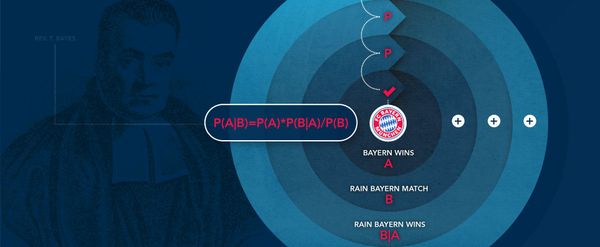# Bayesian analysis and sports betting

#### Bettors often seek out new tools to help refine the process of assigning accurate probabilities to the chances of uncertain events happening. This article discusses how Bayesian Analysis – the theory of an 18th Century English Presbyterian Minister named Thomas Bayes – can help sports bettors gauge the outcome of an event.### The birth of Bayesian analysis

Thomas Bayes was born around 1701 in England, and divided his life between studying matters theological and mathematical. It wasn’t until after his death in 1761 that one of his studies “An Essay towards solving a Problem in the Doctrine of Chances” was submitted to the England’s Royal Society and posthumously acknowledged the significance of his work.

It took, however, until the advent of desktop computers – 200 years later – for Bayes’ work to really be appreciated and gain widespread acceptance. Since then Bayesian analysis has been interpreted and applied in many different spheres such as artificial intelligence. In its simple form the Bayesian approach is arguably the most sensible way to use probability and reasoning to make decisions in the face of uncertainty, and that includes gambling.

It applies an iterative process of assessing what you know about the probability of a future event, and then testing the impact of new evidence as it becomes available.

### The Bayesian analysis Formula

Bayesian analysis can go by many names, ‘Bayesian Inference’, ‘Inverse Probability’, ‘Bayesian Updating’… but at the end of the day it is about a fairly simple formula:

##### P(A|B)= P(A)*P(B|A)/P(B) Probability of A Given B equals Probability of A times Probability of B Given A divided by Probability of B

If you want to know the probability of A when you know that B is also present (given), you can get the answer by multiplying your prior estimation of A (Probability of A) by how much more likely B is when A is present (i.e. P(B|A)/P(B)).

### Using Bayesian analysis to predict the weather

So, suppose you estimate that there is a 30% chance it will rain tomorrow.

And you know that on an average day there is a 50% chance of clouds in the sky.

You also know that the likelihood of clouds is 100% given that rain is 100% (there will always be clouds if there is rain).

You have the following information:

• P(A)= Probability of rain= 30%
• P(B)= Probability of clouds= 50%
• P(B|A)= Probability of clouds given rain= 100%

You wake up in the morning and are blessed with a new piece of information: there are clouds in the sky. You should now perform a Bayesian update on the probability of it raining, Given that there are clouds in the sky.

So, as we recall, P(A|B)=P(A)*P(B|A)/P(B)= chance of rain * chance of clouds given rain/chance of clouds=30%*100%/50%=60%

You can now update your belief about it raining to 60%.

### Bayesian analysis and sports betting

Now, let’s transpose this to a sports betting example. Suppose you are interested in a Bayern Munich match, where you believe they have a 50% chance to win outright. You also know that when they win, it rains 11% of the time, compared to the usual likelihood of rain in a Bayern Munich Match of 10%.

Calculation:

• P(A)= Probability Bayern Munich wins= 50%
• P(B)= Probability of rain in a Bayern Munich match= 10%
• P(B|A)= Probability of rain in a football game when Bayern Munich wins= 11%.

Now, if you receive information about the weather, there is no need to scramble to consider how it will affect the odds. You can, as do many professionals in many fields (including sports betting), perform a Bayesian Update.

If there is rain, you know that P(A|B)=P(A)*P(B|A)/P(B)= 50%*11%/10%= 55%.

Notice that P(B|A)/P(B) is the same as asking “how much More likely is B happening, given A?”- In this case, 11/10 (11%/10%).

Once you know that B is a given, your new estimation of A can change accordingly by simply multiplying them- i.e. P(A)*P(B|A)/P(B).

### Summary

A bettor’s biggest enemy is often himself, with dogmatic adherence to a particular outcome in the face of changing circumstances, a common mistake. Bayesian analysis breaks this habit by allowing and encouraging the constant testing of new evidence against your position, in essence a positive feedback loop of refining your estimates of likelihood of an event.

It isn’t however a mathematical crystal ball, as with any formulas, the GIGO law applies – garbage in, garbage out – but if you have confidence in your assessment of whatever it is your are testing, then the Bayesian approach can unearth sports betting value. And you’ve got an 18th century priest to thank for it.

### MORE: TOP 20 Bookmakers that accept Cryptocurrency >>>

Source: pinnacle.com